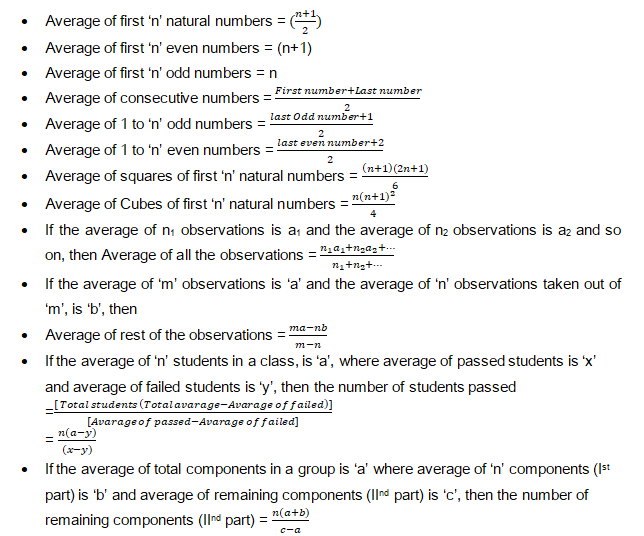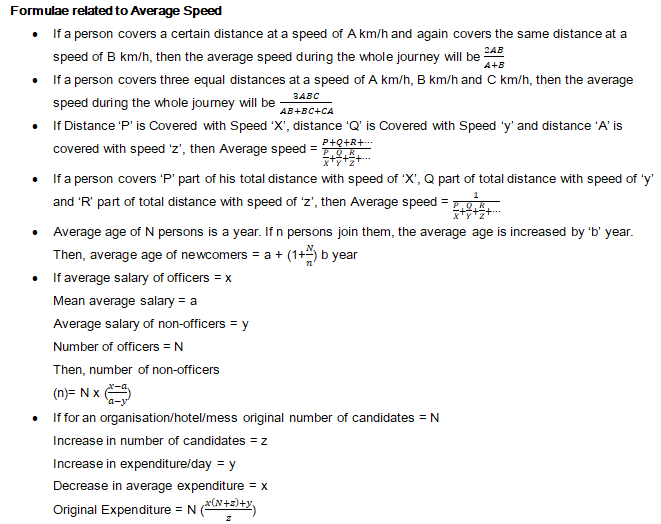# Average

AVERAGES

What is Average?

The result obtained by adding several quantities together and then dividing this total by the number of quantities is called Average. The main term of average is equal sharing of a value among all, where it may share persons or things. We obtain the average of a number using formula that is sum of observations divide by Number of observations.

Average = Sum of Observations/Number of Observations =>S = A × N

Important Points to Remember:

1. a) If all the numbers are increased by ‘a’, then their average will also be increased by ‘a’.
2. b) If all the numbers are decreased by ‘a’, then their average will also be decreased by ‘a’.
3. c) If all the numbers are multiplied by ‘a’, then their average will also be multiplied by ‘a’.
4. d) If all the numbers are divided by ‘a’, then their average will also be divided by ‘a’.

Important Formulae on Averages: